Next: Worked example 7.4: Aerobatic Up: Circular motion Previous: Worked example 7.2: Circular

## Worked example 7.3: Amusement park ride

Question: An amusement park ride consists of a vertical cylinder that spins about a vertical axis. When the cylinder spins sufficiently fast, any person inside it is held up against the wall. Suppose that the coefficient of static friction between a typical person and the wall is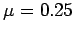. Let the mass of an typical person be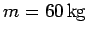, and let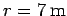be the radius of the cylinder. Find the critical angular velocity of the cylinder above which a typical person will not slide down the wall. How many revolutions per second is the cylinder executing at this critical velocity?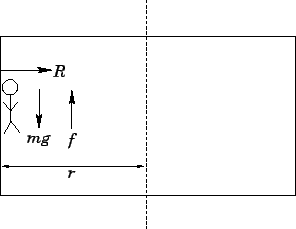Answer: In the vertical direction, the person is subject to a downward force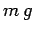due to gravity, and a maximum upward force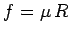due to friction with the wall. Here,is the normal reaction between the person and the wall. In order for the person not to slide down the wall, we require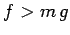. Hence, the critical case corresponds to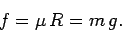In the radial direction, the person is subject to a single force: namely, the reactiondue to the wall, which acts radially inwards. If the cylinder (and, hence, the person) rotates with angular velocity, then this force must provided the acceleration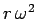towards the axis of rotation. Hence,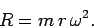It follows that, in the critical case,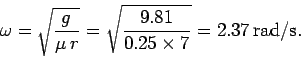The corresponding number of revolutions per second is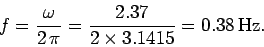Next: Worked example 7.4: Aerobatic Up: Circular motion Previous: Worked example 7.2: Circular
Richard Fitzpatrick 2006-02-02